In the network shown in the figure, each of the resistance is equal to 2 Ω. The resistance between the points A and B is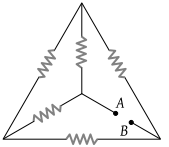(1) 1 Ω

(2) 4 Ω

(3) 3 Ω

(4) 2 Ω

Concept Questions :-

Wheatstone bridge
High Yielding Test Series + Question Bank - NEET 2020

Difficulty Level:

In the arrangement of resistances shown below, the effective resistance between points A and B is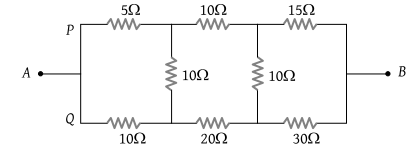(1) 20 Ω

(2) 30 Ω

(3) 90 Ω

(4) 110 Ω

Concept Questions :-

Wheatstone bridge
High Yielding Test Series + Question Bank - NEET 2020

Difficulty Level:

Five resistances are connected as shown in the figure. The effective resistance between the points A and B is :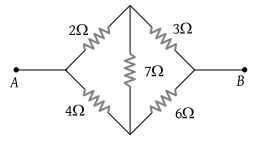(1) $\frac{10}{3}\Omega$

(2) $\frac{20}{3}\Omega$

(3) 15 Ω

(4) 6 Ω

Concept Questions :-

Wheatstone bridge
High Yielding Test Series + Question Bank - NEET 2020

Difficulty Level:

In the given figure, when galvanometer shows no deflection, the current (in ampere) flowing through 5 Ω resistance will be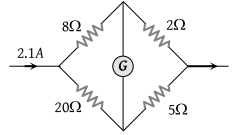(1) 0.5

(2) 0.6

(3) 0.9

(4) 1.5

Concept Questions :-

Wheatstone bridge
High Yielding Test Series + Question Bank - NEET 2020

Difficulty Level:

In the Wheatstone's bridge shown, P = 2 Ω, Q = 3 Ω, R = 6 Ω and S = 8 Ω. In order to obtain balance, shunt resistance across 'S' must be [SCRA 1998]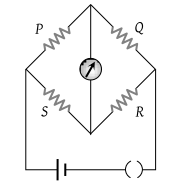(1) 2 Ω

(2) 3 Ω

(3) 6 Ω

(4) 8 Ω

Concept Questions :-

Wheatstone bridge
High Yielding Test Series + Question Bank - NEET 2020

Difficulty Level:

Potential difference between the points P and Q in the electric circuit shown is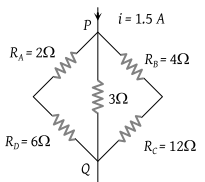(1) 4.5 V

(2) 1.2 V

(3) 2.4 V

(4) 2.88 V

Concept Questions :-

Combination of resistors
High Yielding Test Series + Question Bank - NEET 2020

Difficulty Level:

The current between B and D in the given figure is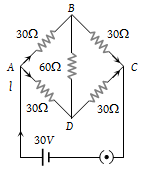(1) 1 amp

(2) 2 amp

(3) Zero

(4) 0.5 amp

Concept Questions :-

Combination of resistors
High Yielding Test Series + Question Bank - NEET 2020

Difficulty Level:

In the given figure, equivalent resistance between A and B will be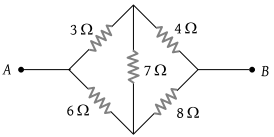(1) $\frac{14}{3}\text{\hspace{0.17em}\hspace{0.17em}}\Omega$

(2) $\frac{3}{14}\text{\hspace{0.17em}\hspace{0.17em}}\Omega$

(3) $\frac{9}{14}\text{\hspace{0.17em}\hspace{0.17em}}\Omega$

(4) $\frac{14}{9}\text{\hspace{0.17em}\hspace{0.17em}}\Omega$

Concept Questions :-

Combination of resistors
High Yielding Test Series + Question Bank - NEET 2020

Difficulty Level:

In a typical Wheatstone network, the resistances in cyclic order are A = 10 Ω, B = 5 Ω, C = 4 Ω and D = 4 Ω for the bridge to be balanced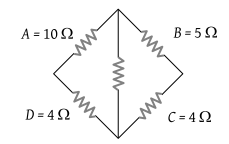(1) 10 Ω should be connected in parallel with A

(2) 10 Ω should be connected in series with A

(3) 5 Ω should be connected in series with B

(4) 5 Ω should be connected in parallel with B

Concept Questions :-

Combination of resistors
High Yielding Test Series + Question Bank - NEET 2020

Difficulty Level:

In the circuit shown in figure, the current drawn from the battery is 4A. If 10 Ω resistor is replaced by 20 Ω resistor, then current drawn from the circuit will be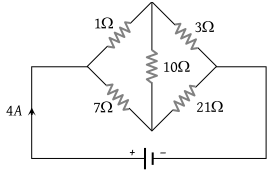(1) 1 A

(2) 2 A

(3) 3 A

(4) 0 A

Concept Questions :-

Combination of resistors